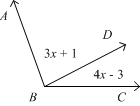We had a great week last week.  A lot of learning took place, students in Geometry brushed up on their Algebra skills, and new Geometry concepts were introduced.  Students had fun trying to figure out patterns, and learning our first postulate, the Segment Addition Postulate!

This week, we will introduce the Angle Addition Postulate.  This lesson will introduce students to angles, how to measure them, how to classify them, and how to incorporate Algebra into them!  We will be working in Chapter 1-4, then moving on to the Midpoint formula and the Distance formula.  Here we will also review the Pythagorean Theorem.  We are looking forward to a great week of learning!